## Surface Area

Surface area is the Area of a given surface. Roughly speaking, it is the amount'' of a surface, and has units of distance squares. It is commonly denoted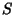for a surface in 3-D, orfor a region of the plane (in which case it is simply called the'' Area).

If the surface is Parameterized usingand, then(1)

whereandare tangent vectors and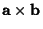is the Cross Product.

The surface area given by rotating the curve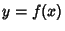from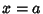to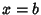about the-axis is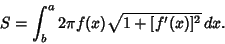(2)

Ifis defined over a region, then(3)

where the integral is taken over the entire surface.

The following tables gives surface areas for some common Surfaces. In the first table,denotes the lateral surface, and in the second,denotes the total surface. In both tables,denotes the Radius,the height,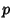the base Perimeter, andthe Slant Height (Beyer 1987).

 SurfaceConeConical Frustum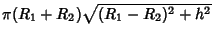Cube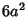Cylinder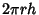Lune (Solid)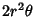Oblate SpheroidProlate SpheroidPyramidPyramidal FrustumSphereTorus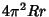ZoneSurfaceCone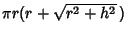Conical FrustumCylinderEven simple surfaces can display surprisingly counterintuitive properties. For instance, the surface of revolution ofaround the x-Axis for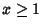is called Gabriel's Horn, and has Finite Volume but Infinite surface Area.